• matlab-S函数编写教程及示例，matlab-S函数入门教程。
• s-function如何编写，此文档讲的比较详细，便于大家系统的学习。另外该文档也有部分示例讲解可以进行操作。
• MATLABS-Function编写指导 ,十分全面，但是具体使用还是要结合众多网友分享的博客，以及自己实操。
• ## Matlab中S-函数的编写

万次阅读 多人点赞 2017-03-01 12:11:13
S-函数使Simulink的功能大大扩充，除Mmatlab外，用户还可以用其他语言（C/C++/FORTRAN/Ada）编写实现算法，很强大的同时也对使用者提出了较高的要求。下面是编写S-函数的整个流程：

0 基础知识

Simulnk仿真分为两步：初始化、仿真循环。仿真是由求解器控制的，求解器主要作用是：计算模块输出、更新模块离散状态、计算连续状态。求解器传递给系统的信息包括：时间、输入和当前状态。系统的作用：计算模块的输出、更新状态、计算状态导数，然后将这些信息传递给求解器。求解器和系统之间的信息传递是通过不同标志来控制的。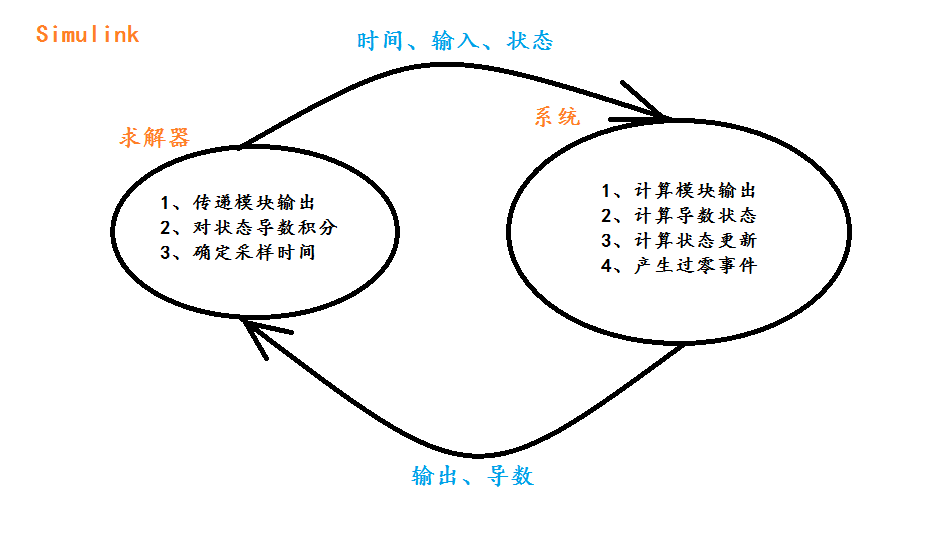、

（2）S-函数控制流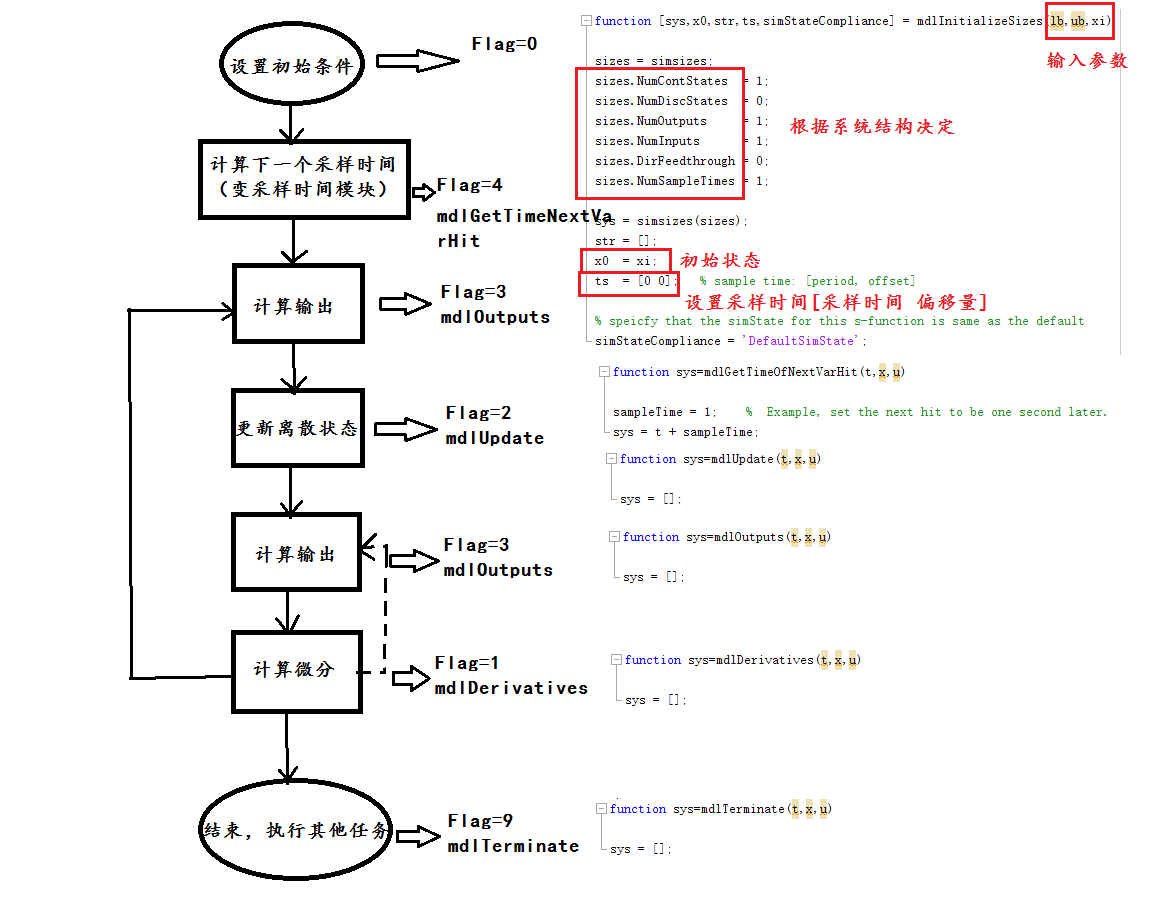（3）S-函数的几个概念

1）  直接馈通
在编写S-函数时，初始化函数中需要对sizes.DirFeedthrough 进行设置，如果输出函数mdlOutputs或者对于变采样时间的mdlGetTimeOfNextVarHit是输入u的函数，则模块具有直接馈通的特性sizes.DirFeedthrough=1;否则为0。

2）  采样时间
仿真步长就是整个模型的基础采样时间，各个子系统或模块的采样时间，必须以这个步长为整数倍。
连续信号和离散信号对计算机而言其实都是采样而来的，只是采样时间不同，连续信号采样时间可认为趋于0且基于微分方程，离散信号采样时间比较长基于差分方程。离散信号当前状态由前一个时刻的状态决定，连续信号可以通过微分方程计算得到。如果要将连续信号离散化还要考虑下信号能否恢复的问题，即香农定理。

采样时间点的确定：下一个采样时间=（n*采样间隔）+ 偏移量，n表示当前的仿真步，从0开始。
对于连续采样时间，ts可以设置为[0 0]，其中偏移量为0；
对于离散采样时间，ts假设为[0.25 0.1]，表示在S-函数仿真开始后0.1s开始每隔0.25s运行一次，当然每个采样时刻都会调用mdlOutPuts和mdlUpdate函数；
对于变采样时间，即离散采样时间的两次采样时间间隔是可变的，每次仿真步开始时都需要用mdlGetTimeNextVarHit计算下一个采样时间的时刻值。ts可以设置为[-2 0]。
对于多个任务，每个任务都可以以不同的采样速率执行S-函数，假设任务A在仿真开始每隔0.25s执行一次，任务B在仿真后0.1s每隔1s执行一次，那么ts设置为[0.25 0.1;1.0 0.1]，具体到S-函数的执行时间为[0 0.1 0.25 0.5 0.75 1.0 1.1…]。
如果用户想继承被连接模块的采样时间，ts只要设置为[-1 0]。

1 S-函数的编写
1.1 S函数的输入输出参数含义

首先打开M-文件的模版函数：function[sys,x0,str,ts,simStateCompliance] = sfuntmpl(t,x,u,flag)
这个是无参的，如果有参数格式为：function[sys,x0,str,ts,simStateCompliance] = sfuntmpl(t,x,u,flag,p1,p2,...)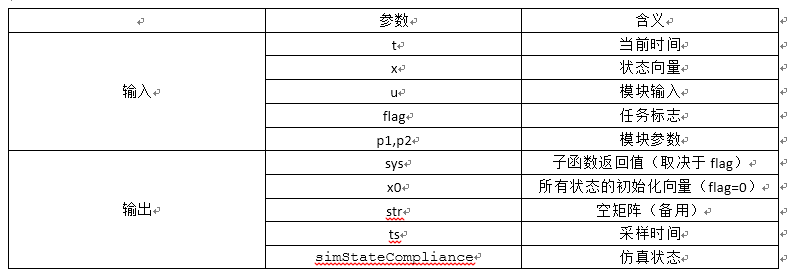1.2 子函数的作用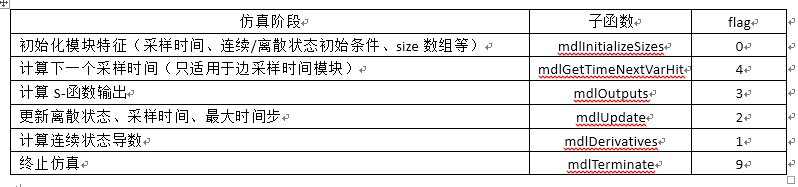（1）
function[sys,x0,str,ts,simStateCompliance]=mdlInitializeSizes
sizes = simsizes;
sizes.NumContStates  = 0;  %连续状态个数
sizes.NumDiscStates  = 0;  %离散状态个数
sizes.NumOutputs     = 0;  %输出个数
sizes.NumInputs      = 0;  %输入个数
sizes.DirFeedthrough = 1;  %是否直接馈通
sizes.NumSampleTimes = 1;  %采样时间个数，至少一个
sys = simsizes(sizes);     %将size结构传到sys中
x0  = [];                     %初始状态向量，由传入的参数决定，没有为空
str = [];
ts  = [0 0];                  %设置采样时间，这里是连续采样，偏移量为0
% Specify the blocksimStateCompliance. The allowed values are:
%    'UnknownSimState', < The defaultsetting; warn and assume DefaultSimState
%    'DefaultSimState', < Same sim state as abuilt-in block
%    'HasNoSimState',   < No sim state
%    'DisallowSimState' < Error out whensaving or restoring the model sim state
simStateCompliance = 'UnknownSimState';


（2）
functionsys=mdlGetTimeOfNextVarHit(t,x,u)
sampleTime = 1;    %  Example, set the next hit to be one secondlater.
sys = t + sampleTime;

（3）
functionsys=mdlOutputs(t,x,u)
sys = [];

（4）
function sys=mdlUpdate(t,x,u)
sys = [];

（5）
functionsys=mdlDerivatives(t,x,u)
sys = [];

（6）
functionsys=mdlTerminate(t,x,u)
sys = [];

2 实例解析
在编写S-函数之前要确定系统是否有状态变量、是连续还是离散状态以及输入输出个数、是否传入参数、采样时间等因素，针对不同的系统进行初始化、编写不同的子函数。
以matlab自带的限制积分函数程序limintm为例，讲解S-函数的编写。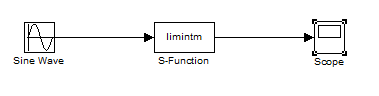S-函数设置：其中传入的参数2,3,2.5分别表示为积分上限、积分下限和初始积分条件。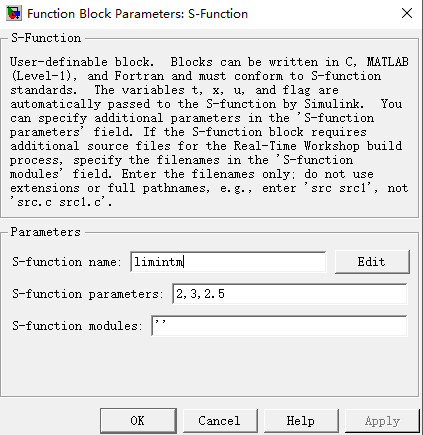输出图形：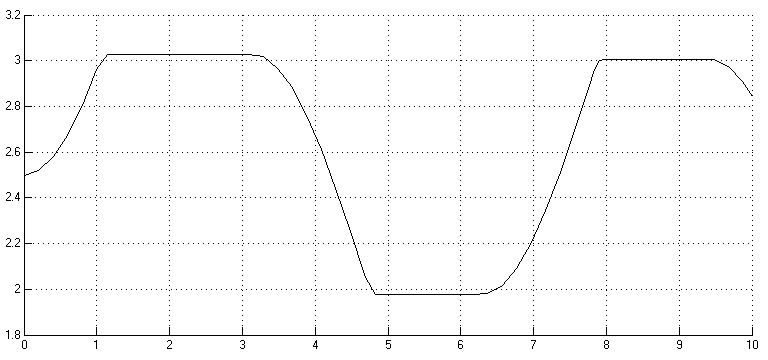S-函数分析：
function [sys,x0,str,ts,simStateCompliance]=limintm(t,x,u,flag,lb,ub,xi)
%传入的三个参数放在后面lb,ub,xi的位置
%LIMINTM Limited integrator implementation.
%   Example MATLAB file S-function implementing a continuous limited integrator
%   where the output is bounded by lower bound (LB) and upper bound (UB)
%   with initial conditions (XI).
%
%   See sfuntmpl.m for a general S-function template.
%

%   Copyright 1990-2009 The MathWorks, Inc.
%   $Revision: 1.1.6.2$

switch flag

%%%%%%%%%%%%%%%%%%
% Initialization %
%%%%%%%%%%%%%%%%%%
case 0
[sys,x0,str,ts,simStateCompliance] = mdlInitializeSizes(lb,ub,xi);

%%%%%%%%%%%%%%%
% Derivatives %
%%%%%%%%%%%%%%%
case 1
sys = mdlDerivatives(t,x,u,lb,ub);

%%%%%%%%%%%%%%%%%%%%%%%%
% Update and Terminate %

%%%%%%%%%%%%%%%%%%%%%%%%
case {2,9}
sys = []; % do nothing

%%%%%%%%%%
% Output %
%%%%%%%%%%
case 3
sys = mdlOutputs(t,x,u);

otherwise
end

% end limintm

%
%=============================================================================
% mdlInitializeSizes
% Return the sizes, initial conditions, and sample times for the S-function.
%=============================================================================
%
function [sys,x0,str,ts,simStateCompliance] = mdlInitializeSizes(lb,ub,xi)

sizes = simsizes;
sizes.NumContStates  = 1;%1个连续状态，即积分状态
sizes.NumDiscStates  = 0;
sizes.NumOutputs     = 1;
sizes.NumInputs      = 1;
sizes.DirFeedthrough = 0;
sizes.NumSampleTimes = 1;

sys = simsizes(sizes);
str = [];
x0  = xi; %积分状态初始条件‘
ts  = [0 0];   % sample time: [period, offset]

% speicfy that the simState for this s-function is same as the default
simStateCompliance = 'DefaultSimState';

% end mdlInitializeSizes

%
%=============================================================================
% mdlDerivatives
% Compute derivatives for continuous states.
%=============================================================================
%
function sys = mdlDerivatives(t,x,u,lb,ub)

if (x <= lb & u < 0)  | (x>= ub & u>0 )
sys = 0;
else
sys = u;
end

% end mdlDerivatives

%
%=============================================================================
% mdlOutputs
% Return the output vector for the S-function
%=============================================================================
%
function sys = mdlOutputs(t,x,u)

sys = x;

% end mdlOutputs


参考：


展开全文• ## matlab中s函数编写心得(转)

千次阅读 多人点赞 2017-04-04 23:33:13
Part I: ... s函数可以用matlab、C、C++、Fortran、Ada等语言来写， 这儿我只介绍怎样用matlab语言来写吧（主要是它比较简单） < xmlnamespace prefix ="o" ns ="urn:schemas-microsoft-com:offic
 Part I:

所谓s函数是system Function的简称, 用它来写自己的simulink模块. s函数可以用matlab、C、C++、Fortran、Ada等语言来写， 这儿我只介绍怎样用matlab语言来写吧（主要是它比较简单） < xmlnamespace prefix ="o" ns ="urn:schemas-microsoft-com:office:office" />

先讲讲为什么要用
s
函数，我觉得用
s
函数可以利用
matlab
的丰富资源，而不仅仅局限于
提供的模块，而用
c
或
c++
等语言写的
s
函数还可以实现对硬件端口的操作，
还可以操作
windows API
等

在matlab的workspace里输入edit sfuntmpl(这是matlab自己提供的s函数模板)，我们看它来具体分析s函数的结构.
1. 函数的函数头
函数的第一行：
function [sys,x0,str,ts]=sfuntmpl(t,x,u,flag) ,
先讲输入与输出变量的含义：
t
是采样时间,
x
是状态变量,
u
是输入(是做成
模块的输入),
flag
是仿真过程中的状态标志(以它来判断当前是初始化还是运行等)
sys
输出根据
flag
的不同而不同(下面将结合
flag
来讲
sys
的含义),
x0
是状态变量的初始值,
str
是保留参数(
mathworks
公司还没想好该怎么用它
,
一般在初始化中将它置空就可以了,
str=[]), ts
是一个
1×2
的向量
, ts(1)
是采样周期
, ts(2)
是偏移量
2. 函数分析
下面结合
sfuntmpl.m
中的代码来讲具体的结构：
switch flag, %
判断
flag
，看当前处于哪个状态
case 0, [sys,x0,str,ts]=mdlInitializeSizes; // 解释说明 flag=0
表示当前处于初始化状态，此时调用函数
mdlInitializeSizes
进行初始化，此函数在
该文件的第
149
行定义. 其中的参数
sys
是一个结构体，它用来设置模块的一些参数，各个参
数详细说明如下
size = simsizes;%
用于设置模块参数的结构体用
simsizes
来生成
sizes.NumContStates = 0; %
模块连续状态变量的个数
sizes.NumDiscStates = 0; %
模块离散状态变量的个数
sizes.NumOutputs = 0; %
模块输出变量的个数
sizes.NumInputs = 0; %
模块输入变量的个数
sizes.DirFeedthrough = 1; %
模块是否存在直接贯通
sizes.NumSampleTimes = 1; %
模块的采样时间个数, 至少是一个
sys = simsizes(sizes); %
设置完后赋给
sys
输出

举个例子，考虑如下模型:
dx/dt=fc(t,x,u)
也可以用连续状态方程描述：
dx/dt=A*x+B*u x(k+1)=fd(t,x,u)
也可以用离散状态方程描述：
x(k+1)=H*x(k)+G*u(k) y=fo(t,x,u)
也可以用输出状态方程描述：
y=C*x+D*u
设上述模型连续状态变量、离散状态变量、输入变量、输出变量均为
1
个，我们就只需改上面那一段代码为(
一般连续状态与离散状态不会一块用, 我这儿是为了方便说明):
sizes.NumContStates=1;sizes.NumDiscStates=1;sizes.NumOutputs=1;sizes.NumInputs=1;
其他的可以不变, 继续在
mdlInitializeSizes
函数中往下看：
x0 = []; %
状态变量设置为空，表示没有状态变量，以我们上面的假设，可改
为
x0=[0,0](
离散和连续的状态变量我们都设它初值为
0) str = []; %
保留参数, 置
[]
就可以了, 没什么用
ts = [0 0]; %
采样周期设为
0
表示是连续系统, 如果是离散系统在下面的
mdlGetTimeOfNextVarHit
函数中具体介绍

case 1, sys=mdlDerivatives(t,x,u); // flag=1
表示此时要计算连续状态的微分, 即上面提到的
dx/dt=fc(t,x,u)
中的
dx/dt,
找到193行的
函数mdlDerivatives
,
如果设置连续状态变量个数为
0
, 此处只需
sys=[]
就可以了,
按我们上述讨论的那个模型, 此处改成
sys=fc(t,x(1),u)
或
sys=A*x(1)+B*u,
我们这儿
x(1)
是连续状态变量, 而
x(2)
是离散的,
这儿只用到连续的, 此时的输出
sys
就是微分

case 2, sys=mdlUpdate(t,x,u); // flag=2
表示此时要计算下一个离散状态, 即上面提到的
x(k+1)=fd(t,x,u),
找到
mdlUpdate
函数,
它这儿
sys=[]
表示没有离散状态, 我们这儿可以改成
sys=fd(t,x(2),u)
或
sys=H*x(2)+G*u;%sys
即为
x(k+1)
case 3, sys=mdlOutputs(t,x,u); //
flag=3
表示此时要计算输出, 即
y=fo(t,x,u),
找到218行的
mdlOutputs
函数.
如果
sys=[]
表示没有输出, 我们改成
sys=fo(t,x,u)
或
sys=C*x+D*u %sys
此时为输出
y
case 4, sys=mdlGetTimeOfNextVarHit(t,x,u); // flag=4
表示此时要计算下一次采样的时间, 只在离散采样系统中有用
(
即上文的
mdlInitializeSizes
中提到的
ts
设置
ts(1)
不为
0),
连续系统中只需在
mdlGetTimeOfNextVarHit
函数中写上
sys=[].
这个函数主要用于变步长的设置, 具体实现大家可以用
edit vsfunc
看
vsfunc.m
这个例子

case 9, sys=mdlTerminate(t,x,u); // flag=9
表示此时系统要结束，一般来说写上在
mdlTerminate
函数中写上
sys=[]
就可, 如
果你在结束时还要设置什么，就在此函数中写
完了.
Part II 此外, s函数还可以带用户参数, 下面给个例子, 它和simulink下的gain模块功能一样 function [sys,x0,str,ts] = sfungain(t,x,u,flag,gain) switch flag, case 0, sizes = simsizes; sizes.NumContStates = 0; sizes.NumDiscStates = 0; sizes.NumOutputs = 1; sizes.NumInputs = 1; sizes.DirFeedthrough = 1; sizes.NumSampleTimes = 1; sys = simsizes(sizes); x0=[]; str=[]; ts=[0,0]; case 3, sys=gain*u; case {1,2,4,9}, sys = []; end 做好了s函数后, simulink --> user-defined function下拖一个S-Function到你的模型, 就可以用了. 在simulink --> user-defined function还有个s-Function Builder, 他可以生成用c语言写的s函数. 或者在matlab的workspace下打sfundemos, 可以看到很多演示s函数的程序

Part III

展开全文• MATLAB编写函数文件的实例 1.在M文件编辑器中，编写“ssort”函数的代码 函数代码如下： function out=ssort(a) %ssort程序代码按照升序排列数据 %Define variables: %a input array to sort %ii index variable %...
MATLAB编写函数文件的实例 1.在M文件编辑器中，编写“ssort”函数的代码 函数代码如下：

function out=ssort(a) %ssort程序代码按照升序排列数据 %Define variables: %a input array to sort %ii index variable %nvals number of values in “a” %out sorted output array %temp temp variable for swaping nvals=size(a,2); for ii=1:nvals-1 iptr=ii; for jj=ii+1:nvals if a(jj)<a(iptr) iptr=jj; end end if ii~=iptr temp=a(ii); a(ii)=a(iptr); a(iptr)=temp; end

end out=a;

2.保存程序代码 3.编写“test_sort”脚本文件 脚本文件代码如下：

nvals=input(‘Enter number of the numbers to sort:’); 1 array=zeros(1,nvals); for ii=1:nvals string=[‘Enter value’ int2str(ii) ‘:’]; array(ii)=input(string); end sorted=ssort(array); fprintf(’\n sorted data:\n’); for ii=1:nvals fprintf(’%8.4f\n’,sorted(ii)); end

4.保存程序代码 5.运行代码 在MATLAB命令窗口中输入“test_sort”,按下回车键，根据所得的结果依次进行。

作者：果果。 来源：CSDN 原文：https://blog.csdn.net/qq_44877251/article/details/89052791 版权声明：本文为博主原创文章，转载请附上博文链接！
展开全文• [s函数实例]（https://blog.csdn.net/qq_34317499/article/details/81044757） S函数代码如下： function [sys,x0,str,ts]=zhai(t,x,u,flag,k,b) switch flag case 0 [sys,x0,str,ts]=mdlInitial...
这是个简单的例子，注：此博客参考

[s函数小实例]（https://blog.csdn.net/qq_34317499/article/details/81044757）

S函数代码如下：
function [sys,x0,str,ts]=zhai(t,x,u,flag,k,b)
switch flag
case 0
[sys,x0,str,ts]=mdlInitializeSizes; %初始化
case 3
sys=mdlOutputs(t,x,u,k,b);          %计算输出量
case {1,2,4,9}
sys=[];
otherwise                               %出错处理
error(num2str(flag))
end
function [sys,x0,str,ts]=mdlInitializeSizes()
sizes=simsizes;
sizes.NumContStates=0;   %无连续状态
sizes.NumDiscStates=0;    %无离散状态
sizes.NumOutputs=1;            %有一个输出量
sizes.NumInputs=1;         %有一个输入信号
sizes.DirFeedthrough=1;   %输出量中含有输入量
sizes.NumSampleTimes=1;   %单个采样周期
sys=simsizes(sizes);
%给其他返回参数赋值
x0=[];                   %设置初始状态为零状态
str=[];                    %将str变量设置为空字符串
ts=[-1,0];                 %假定继承输入信号的采样周期
function sys=mdlOutputs(t,x,u,k,b)
sys=k*u+b;

Simulink模型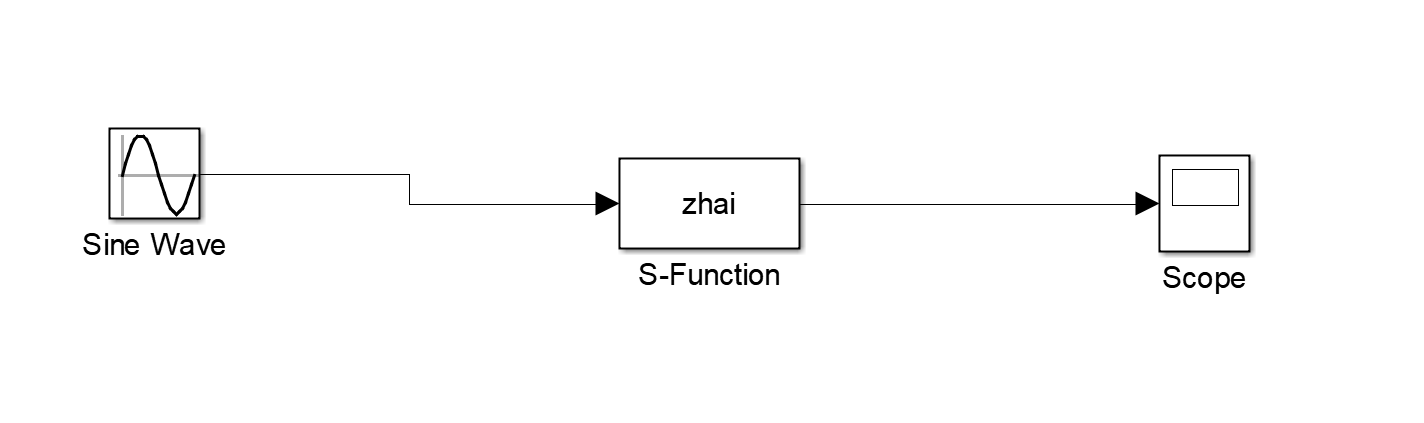S函数参数设置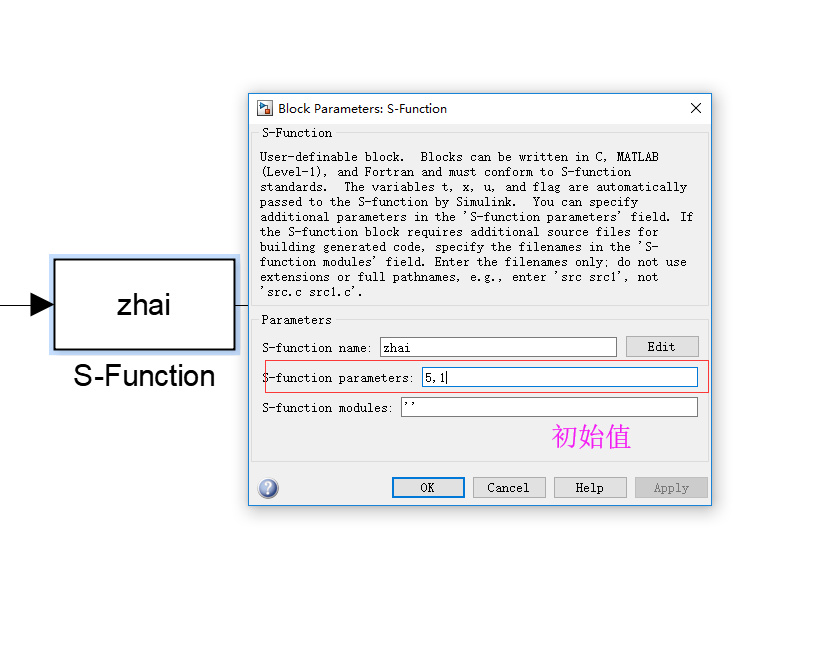其中 ：正弦波为输入，别忘了设置初始值（一开始我忘了，找了好久原因），这个是一个输入，一个输出的例子
仿真结果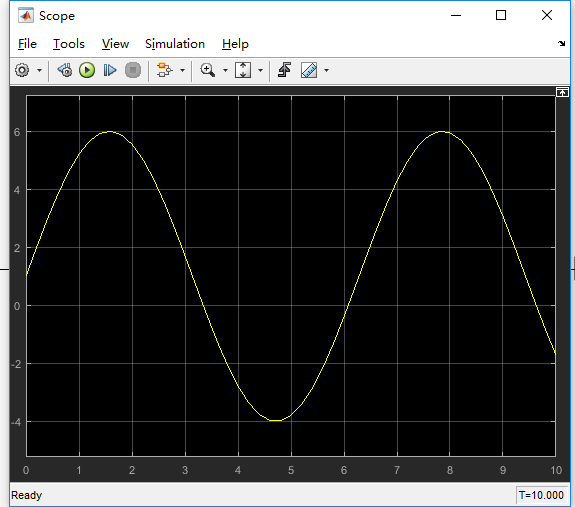输入为2个及以上变量时，只需修改
sizes.NumInputs=2;         %有二个输入信号

%%%%%%%%
function sys=mdlOutputs(t,x,u,k,b)
sys=k*u(1)+b*u(2);

效果图如下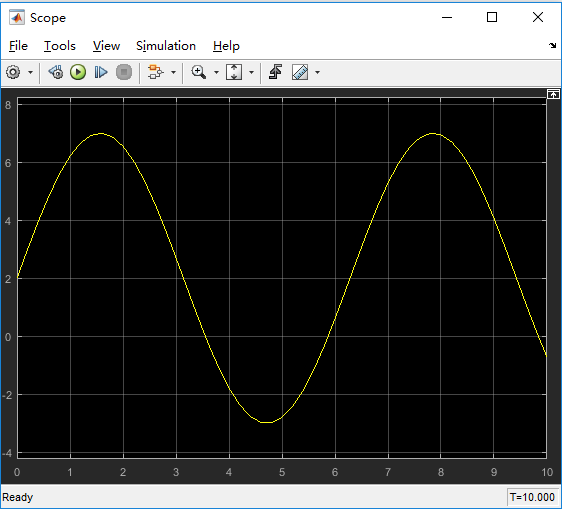展开全文• 设计实例1;设计实例1;设计实例1;设计实例1;设计实例1;设计实例1;设计实例1;设计实例2;设计实例2;设计实例2;设计实例2;设计实例2;设计实例2;设计实例2;设计实例2;Slide 20;Slide 21;第1部分 MATLAB基础;1.1 MATLAB...
• ## MatlabS函数编写

千次阅读 2009-04-10 19:20:00function fortran 语言 任务
• ## matlab中S函数的概念及使用

万次阅读 多人点赞 2018-04-08 21:03:31simulink
• ## MATLAB分段函数及应用实例

万次阅读 多人点赞 2018-02-24 22:24:08
简单实例：用matlab画分段函数 当x&lt;0时 y=5*sin(x); 当x&gt;=0且x&lt;=5时 y=x^2; 当x&gt;5时 y=(8-x)^2+16.x=-5:0.001:10; y=5*sin(x).*(x&lt;0)+x.^2.*(x&gt;=0 &amp; x&lt...速度曲线
• ## MATLAB GUI设计快速入门实例

万次阅读 多人点赞 2019-11-27 10:47:15
为了实现课题的一个功能，需要设计一个图形界面，因此使用matlab GUIDE进行设计，但前期没有接触过，因此通过实例练习，理解MATLAB gui设计相关知识。 一、几个GUI设计需要了解的知识 1、进入GUI 设计界面：在命令行...
• Matlab允许你使用以下五种方式之一来实现S函数： 　 A Level-1 M-file S-function provides a simple M interface to interact with a small portion of the S-function API. Level-2 M-file S-functions supersede...
• 本代码主要利用MATLAB工具实现MATLAB——完整m文件实例，简单明了，易于理解
• 工程优化设计中常常用到的惩罚函数法的Matlab实现的源程序代码
• ## MATLAB函数速查手册

千次阅读 多人点赞 2018-03-25 09:06:26matlab
• s函数官方编程手册（英文），主要包括S-function的介绍、详细方法、内部原理介绍、使用MATLAB、C、C++、Fortran等编写S函数、S函数编写实例等。
• function [m]=intgauss(n) % n代表所求节点的个数 syms x for i=1:n for j=1:n y(i,j)=int(log(x)*x(i-1)*x(n-j),0,1); % 积分的权函数不同则log(x)会改变 ...h=h’ % h表示权函数与正交函数与w(x)
• ## MatlabS-function 使用总结

万次阅读 多人点赞 2019-05-24 09:45:03
• ## S-函数的编写规则

千次阅读 2019-05-08 16:37:45
• Simulink 仿真+S函数例子 S 函数代码如下： function [sys,x0,str,ts,simStateCompliance] = Eg3_7_SFunction(t,x,u,flag) switch flag case 0 % 初始化 [sys,x0,str,ts,simStateCompliance] = ...
• 3.2.2 M文件S-函数编写示例 30 3.3 C语言S-函数 46 3.3.1 C语言S-函数简介 46 3.3.2 C语言S-函数编写示例 51 3.4 C 语言S-函数 60 第4章 信源和信宿 66 4.1 信源 66 4.1.1 压控振荡器 66 4.1.2...
• 比较二次函数值的大小是二次函数图像与性质应用的重要题型之一，是中考的热点。要熟练准确地解决这类问题，同学们要理解二次函数的增减性、能画出图像的大致位置，会确定对称轴，还要掌握解决这类问题的一般方法和...
• 本资源包含基于Matlab的使用sum方法计算矩阵、数组、向量元素总和 包含 实例1：计算向量元素...本资源配套CSDN博客“Matlab函数学习---sum函数(计算矩阵、数组和向量元素总和)”，可前往查看具体原理和实现效果！！！
• 基于Matlab的图形用户界面（GUI）设计，该算法实现了一元二次函数y=ax^2+bx+c的图形绘制，其中a、b、c为可输入参数，能够多次绘制函数图像，也能叠加绘制函数图像等...

# matlabs函数编写实例matlab 订阅